Processing ......FreeComputerBooks.com Links to Free Computer, Mathematics, Technical Books all over the World

A Handbook of Statistical Analyses Using R
Top Free C++ Books 🌠 - 100% Free or Open Source!
• Title: A Handbook of Statistical Analyses Using R
• Author(s) Brian S. Everitt, Torsten Hothorn
• Publisher: Chapman and Hall/CRC; 1 edition (February 17, 2006)
• Paperback: 304 pages
• eBook: PDF (Draft, 207 pages, 2.8 MB)
• Language: English
• ISBN-10: 1584884509
• ISBN-13: 978-1584884507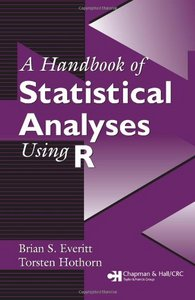Book Description

Doing for R what Everitt's other Handbooks have done for S-PLUS, STATA, SPSS, and SAS, A Handbook of Statistical Analyses Using R presents straightforward, self-contained descriptions of how to perform a variety of statistical analyses in the R environment.

From simple inference to recursive partitioning and cluster analysis, eminent experts Everitt and Hothorn lead you methodically through the steps, commands, and interpretation of the results, addressing theory and statistical background only when useful or necessary.

They begin with an introduction to R, discussing the syntax, general operators, and basic data manipulation while summarizing the most important features. Numerous figures highlight R's strong graphical capabilities and exercises at the end of each chapter reinforce the techniques and concepts presented.

All data sets and code used in the book are available as a downloadable package from CRAN, the R online archive.

A Handbook of Statistical Analyses Using R is the perfect guide for newcomers as well as seasoned users of R who want concrete, step-by-step guidance on how to use the software easily and effectively for nearly any statistical analysis.

• N/A

Reviews, Ratings, and Recommendations: Related Book Categories: Read and Download Links:Similar Books:
•Learning Statistics with R (Daniel Navarro)

This book takes you on a guided tour of software development with R, from basic types and data structures to advanced topics like closures, recursion, and anonymous functions. No statistical knowledge is required.

•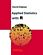Applied Statistics with R (David Dalpiaz)

This book provides an integrated treatment of statistical inference techniques in data science using the R Statistical Software. It provides a much-needed, easy-to-follow introduction to statistics and the R programming language.

•Statistics with R (Vincent Zoonekynd)

Provides an elementary-level introduction to R, targeting both non-statistician scientists in various fields and students of statistics via code examples with liberal commenting of the code and the output, from the computational as well as the statistical viewpoint.

•Introduction to Probability and Statistics Using R (G. Jay Kerns)

This is a textbook for an undergraduate course in probability and statistics, covers various basic and advanced statistical procedures, from correlation and graph creation to analysis of variance, regression, non-parametric tests, and more.

•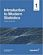Introduction to Modern Statistics (Mine Çetinkaya-Rundel, et al.)

This book puts a heavy emphasis on exploratory data analysis and provides a thorough discussion of simulation-based inference using randomization and bootstrapping, followed by a presentation of the related Central Limit Theorem based approaches.

•Foundations in Statistical Reasoning (Pete Kaslik)

This book is designed for students taking an introductory statistics class. The emphasis throughout the entire book is on how to make decisions with only partial evidence. It focuses on the thought process.

•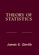Theory of Statistics (James E. Gentle)

This book is directed toward students for whom mathematical statistics is or will become an important part of their lives. It covers classical likelihood, Bayesian, and permutation inference; an introduction to basic asymptotic distribution theory; and modern topics.

•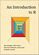An Introduction to R (Alex Douglas, et al.)

The main aim of this book is to help you climb the initial learning curve and provide you with the basic skills and experience (and confidence!) to enable you to further your experience in using R.

•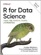R for Data Science: Visualize, Model, Transform, Tidy, Import

This book teaches you how to do data science with R: You'll learn how to get your data into R, get it into the most useful structure, transform it, visualize it and model it, how data science can help you work with the uncertainty and capture the opportunities.

•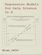Regression Models for Data Science in R (Brian Caffo)

The book gives a rigorous treatment of the elementary concepts of regression models from a practical perspective. The ideal reader for this book will be quantitatively literate and has a basic understanding of statistical concepts and R programming.

•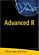Advanced R (Florian Prive)

This book aims at giving a wide understanding of many aspects of R. Combining detailed explanations with real-world examples and exercises, this book will provide you with a solid understanding of both statistics and the depth of R's functionality.

•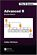Advanced R, Second Edition (Hadley Wickham)

This book helps you understand how R works at a fundamental level. Designed for R programmers who want to deepen their understanding of the language, and programmers experienced in other languages to understand what makes R different and special.

•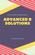Advanced R Solutions (Malte Grosser, et al)

This book offers solutions to the exercises from Advanced R, 2nd Edition by Hadley Wickham. It is work in progress and under active development. The 2nd edition of Advanced R is in print now and we hope to provide most of the answers.

•An Introduction to Statistical Learning: with Applications in R

It provides an accessible overview of the field of statistical learning, an essential toolset for making sense of the vast and complex data sets that have emerged in fields ranging from biology to finance to marketing to astrophysics in the past twenty years.

Book Categories
 :All CategoriesTop Free BooksRecent BooksMiscellaneous BooksComputer EngineeringComputer LanguagesComputer ScienceData Science/DatabasesJava and Java EE (J2EE)Linux and UnixMathematicsMicrosoft and .NETMobile ComputingNetworking and CommunicationsSoftware EngineeringSpecial TopicsWeb Programming
Other Categories